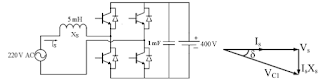# Gate Electrical Answer Key 2016

Q.47 A DC-DC boost converter, as shown in the figure below, is used to boost 360V to 400 V, at a power of 4 kW. All devices are ideal. Considering continuous inductor current, the rms current in the solid state switch (S), in ampere, is _________.Q.48 A single-phase bi-directional voltage source converter (VSC) is shown in the figure below. All devices are ideal. It is used to charge a battery at 400 V with power of 5 kW from a source Vs = 220 V (rms), 50 Hz sinusoidal AC mains at unity p.f. If its AC side interfacing inductor is 5 mH and the switches are operated at 20 kHz, then the phase shift (δ) between AC mains voltage (Vs) and fundamental AC rms VSC voltage (VC1), in degree, is _________.Q.49Q.50 A second-order real system has the following properties: a) the damping ratio =0.5 and undamped natural frequency Wn=10 rad/s, b) the steady state value of the output, to a unit step input, is 1.02. The transfer function of the system is

Please report if you find any errors in the questions.
Keep watching the space